### Reflection

1. What is specular reflection?

2. What is diffuse reflection?

3. What type of reflection makes objects appear shiny?

4. What does the law of reflection say?

5. Draw a light ray reflecting off of a surface and label the angle of incidence and the angle of reflection.

1. ANSWER: Specular reflection means that parallel light rays hitting a surface remain parallel after reflection.

2. ANSWER: During diffuse reflection each individual light ray still follows the law of reflection, but because the surface that these light rays hit is not flat on a microscopic (or even macroscopic) level, incoming parallel light rays don't remain parallel after reflection.

4. ANSWER: The angle at which a light ray hits a surface, or the angle of incidence, is equal to the angle at which the light ray is reflected, or the angle of reflection.

5. ANSWER: This should be similar to the diagram in the mirror section (link to it).

### Mirrors

Plane Mirrors:

1. Where is the focal point, F, of a plane mirror?

2. You are given the incident angle, θi, of a plane mirror of 30°. What is θr?

Concave Mirrors:

3. It's Mother's Day and we want to surprise mom with a cosmetic mirror for her bathroom. We go to Home Depot and it turns out that cosmetic mirrors come with different curvatures. We don't want Mom to have to climb onto the vanity to apply her make-up, nor would applying make-up be efficient if she has to stand all the way across the room from her new mirror. Ideally, she wants to stand at the edge of the vanity to make herself presentable. If the vanity is just under 1 meter deep what radius of curvature, r, should the mirror have for her to see a right side up, magnified version of herself while standing in front of it?

(A) 2m
(B) 0.5m
(C) 1m
(D) Next year we're sticking with flowers.

4. What would Mom see if we got her the mirror with a 0.5 m radius of curvature?

5. Let's say we messed up and got that 0.5 m radius mirror for her. What would she have to do to still see a right side up, magnified version of herself?

6. What if we wanted Mom to see a magnified, upside down version of herself? What radius of curvature would your mirror have to have?

1. Answer:A plane mirror doesn't have a focal point and the light rays are never focused by a plane mirror.

2. ANSWER: For a plane mirror, θi = θr, so θr = 30°.

3. Answer: Although (D) may also be correct, the mathematical answer is (A). Remember, a concave mirror only generates a magnified, right side up image, when an object is placed between the focal point, F, and the mirror surface. Since Mom's vanity is just under 1 m from the bathroom wall, she would want a mirror that has the focal point 1m from the wall, or in other words a focal length, f, of 1 m. The focal length has a simple relationship to the radius of curvature, r, of the mirror: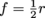or r = 2f. Since f = 1 m, r = 2 m.

4. Answer: In this case, f = 0.25 m. Since the radius of curvature is r = 0.5 m, the center of curvature is 0.5 m in front of the mirror. At the edge of the vanity, your mom would be 1 m away from the mirror and beyond the center of curvature of the mirror. She would be trying to apply her makeup while seeing a tiny, upside down version of herself. We could use the equation to check this, or sketch a ray diagram.

5. Answer: We calculate the focal length f = 0.25 m. To see a useful version of herself she has to place her face between the focal point and the mirror. Since the focal distance of the mirror is 0.25 m, Mom would have to climb onto the vanity and pretty much smoosh her face up against the mirror to see herself right side up and magnified.

6. Answer: To see a magnified, upside down version of herself at a distance of 1 m from the mirror, she would have to be standing between the focus, F, and the center of curvature, C, of the mirror. In other words, the mirror would have to have a focal length smaller than 1 m, and a radius of curvature larger than 1 m. Any mirror that satisfies these requirements would work, so there are clearly many correct answers to this question. For one example, let's arbitrarily set the focal length f to 0.75 m. Then r = 2f = 2(0.75 m) = 1.5 m. At the edge of the vanity, at 1 m, Mom would be right in between the center of curvature and the focal point of the mirror.

Convex Mirrors:

7. Draw a ray diagram showing what sort of image is generated by a convex mirror. We didn't work through this in this section, but just do what we did for the concave mirrors and we'll be golden. HS-PS4-1, Phys-4.f, Hard, 4

8. What kind of an image is created in (7)?

(A) A real image
(B) A focal point
(C) A virtual image
(D) Can we just go back to talking about the flower's we're getting my mom next year?

7. Answer: Draw the same three rays as usual, all coming from the top of the object. Avoid the common mistake of drawing rays from both the top and the bottom of the image: the bottom of the image remains on the center axis as long as that's where it starts. The only time we draw rays from all possible corners of an object is when we try to determine what the virtual object generated by a plane mirror looks like.

Each ray will be reflected according to the law of reflection and you will see that the two rays do not converge. To make them converge you have to extend them into the mirror. The top of the image generated by the convex mirror is where the three extended rays intersect: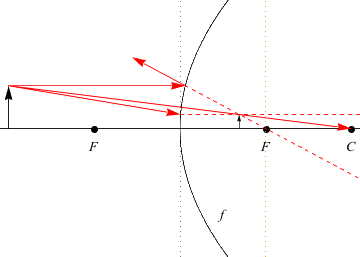8. Answer: It's a virtual image. All convex mirrors are diverging mirrors. That is, they spread light rays out rather than focus them, or bring them together. The actual light rays never touch the image.

### Refraction

1. The refractive index of water is 1.3. The refractive index of a diamond is 2.4. How much faster does light travel in water than in a diamond?

2. Calculate by how much a light beam is bent away from the normal when it travels from a diamond, with n = 2.4, into water, which has a refractive index n = 1.3, if the light beam hits the diamond water interface at an angle θ1 = 33° with respect to the normal.

3. Light travels from a medium with a refractive index of n1 = 2.5 into a slab of lucite with a refractive index n1 = 1.5. It hits the Lucite with an incident angle θ1 = 20°. The light is refracted, continues through the box of Lucite and out the other side of the box back into the medium with a refractive index of 2.5. At what angle does the light exit the box?

4. What is the speed of light in lucite if lucite's index of refraction is 1.5?

5. Light leaves a medium with a refractive index of 1.5 and enters a medium of unknown refractive index. The angle of incidence, θ1, is 35°, and θ2 is 25°. What is the refractive index of the second medium?

1. ANSWER: Step one to answering this question is to determine the speed of light in water and then the speed of light in a diamond using the equation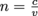. In this scenario we are given "n" and want to solve for "v" so we rearrange the equation.

Now, we can solve for the velocity of light in water, vwater, and the velocity of light in a diamond, vdiamond: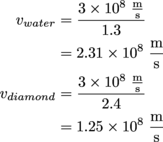But we're not done yet. The question asked how much faster does light travel through water than through a diamond so we have to subtract the speed of light in a diamond, vdiamond, from the speed of light in water, vwater, which is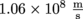.

2. Answer: It's always a good idea to quickly sketch a diagram for problems like this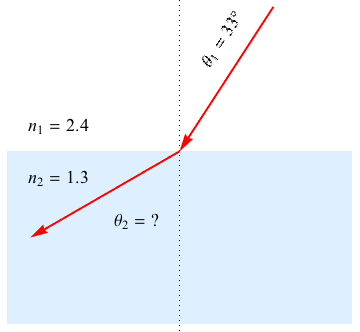Since this is a question about refraction, we will use Snell's law: n1sin θ1 = n2sin θ2 to solve for θ2: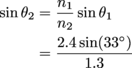sin θ2 = 1
θ2 = sin-1 (1)
θ2 = 90°

Let's redraw our ray diagram.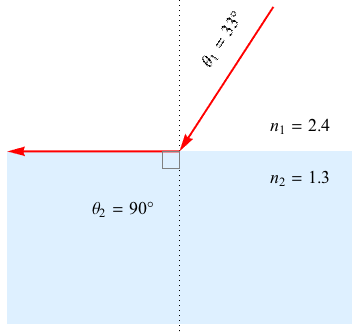As we can see, the light beam bends such that it travels along the surface of our diamond. It's trapped at the diamond-water interface. The angle θ1 when θ2 = 90°, is called the critical angle, θc. At incident angles greater than the critical angle, so in our example at θ1 > 33°, no light will be refracted. All light will be reflected. This is called total internal reflection.

3. ANSWER: With these types of questions you always want to draw out what is happening and fill in all of the values you know. It is easy to get overwhelmed by light moving into the block and then back out. Just take a deep breath and take it one step at a time: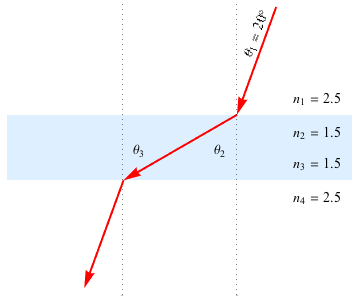Step 1: We can calculate θ2 using Snell's law, n1sin θ1 = n2sin θ2
n2sin θ2 = n1sinθ1
n2
sin θ2 = (2.5) sin 20°
n2sin θ2 = 0.85
sin θ2 = 0.57
θ2 = 35°
So far so good. A quick concept check affirms that our answer makes sense. Since n1 > n2, θ1 should be smaller than θ2.

Step 2: The next part doesn't even involve math. Because the two normals in our diagram are parallel, geometry tell us that θ3 = θ2 by alternate interior angles.

Step 3: And now that we know θ3, we're back to Snell's law again.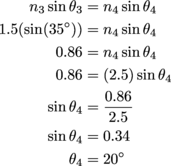Based on the diagram we drew, this answer makes sense. θ4 should be smaller than θ3. Furthermore, θ4 = θ1 as it should because the light reenters the same medium in which it started and should continue in the same direction, albeit offset from its initial path because of the refraction.

4. ANSWER: To answer this question we use the definition of the index of refraction and rearrange.
The velocity of light in Lucite is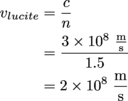5. ANSWER: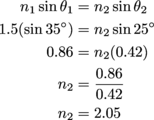### Lenses

1. Why don't we use concave lenses in a camera to fit the Eiffel Tower on our one-inch film?

2. Draw a ray diagram to see what happens when you put an image a distance greater than 2F from a concave lens.

3. What type of an image is formed in Question 2?
(a) A real image that is upside down and magnified
(b) A real image that is right side up and smaller
(c) A virtual image that is right side up and magnified
(d) A virtual image the is right side up and smaller

4. Willebrord Snellius is trying out for the football team. Unfortunately, Willebrord is only 1.5 m tall and he is so skinny that we are all quite certain that the running back would knock him over just by looking at him. But all of us like Willebrord and think that he should, at least, be allowed to try out rather than be run off of the field by the coach before he has a chance to prove himself. What type of lens, concave or convex, would we want to replace the coach's sunglasses with to make Willebrord seem larger?

5. There is one problem with this plan. Concave lenses magnify differently depending on where on object is placed relative to the lens. If the coach meets Willebrord from too close or too far away, Willebrord might end up seeming upside down. Or worse yet, upside down and even smaller! To answer the next set of questions, first draw what happens when Willebrord is standing (a) between the coach and the focal point of the lens; (b) a distance greater than twice the focal length away from the coach; and (c) between the focal point F and 2F. This is a bit of work but trust us, the more we practice ray diagrams, the easier it gets.
So based on our diagrams, where would Willebrord have to stand relative to the coach to look upside down but at least still bigger?

6. Where would Willi really not want to stand? What position would make him look not only upside down but also smaller?

7. What is the best spot for Willebrord?

8. We decide to get those glasses for the coach. There are lots of different options: the different lenses differ quite a bit in focal length. A reasonable distance for Willebrord to be meeting the coach from seems to be from 15 meters away so we decide on a lens with a focal distance of 10 meters. How tall will Willebrord look to the coach when he is 15 meters away from him? Hint: We need to use both the thin lens and the magnification equation.

1. Answer: There are two reasons. For one, concave lenses generate virtual images. There are no light rays where the image is formed so the image can't be captured on film by using that kind of lens. The second reason is more practical. The image is formed on the same side of the lens as the object that you are trying to take a picture of. Where could we put the film?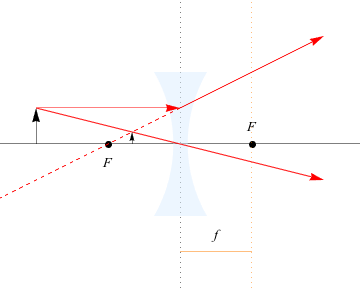3. Answer: (d) A virtual image that is right side up and smaller.

4. Answer: Concave lenses don't enlarge things so it would have to be a convex lens.

5. Answer: Between the focal point, F, and 2F of the lens in the coach's sunglasses.

6. Answer: A distance greater than twice the focal length of the lens in the coach's glasses.

7. Answer: Between the focal point, F, and the coach.

The magnification equation will tell us how much taller Willi will be. Here is it again.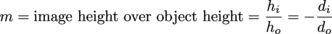We know the object height, Willi is 1.5 m tall. We also know the object distance, do = 15 m.

We next determine whether the object distance and height will be positive or negative. To do this, we need to figure out whether we are forming a real or a virtual image. With the information we have been given, we can do that.

We know that since f = 10 m, 2F is 20 meters away from the coach. Since Willi will be standing 15 m away from the coach, he will be standing between the focal point F and 2F. We just determined by ray diagram that when an object is between F and 2F, convex lenses generate a real, upside down, magnified image, so do is positive.

We're still missing either the image height or distance to be able to use the magnification equation.

Since we know that the focal length of the lens is 10 m, and that Willi will be an object distance of 15 m away from the coach, we next use the thin lens equation to determine the image distance from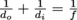Solving this equation for di we get an object distance of 30m.
We can now use the magnification equation to see how much bigger Willi will be: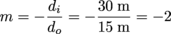Willi will appear 1.5 m × -2 = -3 m tall. And see that negative sign? That's important: it means that the object will be magnified BUT upside down! Which we already knew based on where Willi was standing relative to the focal point of the lens. Maybe we didn't think this through so well when we bought those lenses.